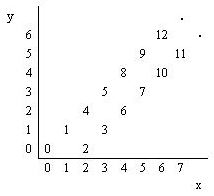poj1663,

## Problem:

Number Steps
 Time Limit: 1000MS Memory Limit: 10000K Total Submissions: 10817 Accepted: 5763
Description
Starting from point (0,0) on a plane, we have written all non-negative integers 0,1,2, ... as shown in the figure. For example, 1, 2, and 3 has been written at points (1,1), (2,0), and (3, 1) respectively and this pattern has continued.You are to write a program that reads the coordinates of a point (x, y), and writes the number (if any) that has been written at that point. (x, y) coordinates in the input are in the range 0...5000.
Input
The first line of the input is N, the number of test cases for this problem. In each of the N following lines, there is x, and y representing the coordinates (x, y) of a point.
Output
For each point in the input, write the number written at that point or write No Number if there is none.
Sample Input
```3
4 2
6 6
3 4```
Sample Output
```6
12
No Number```
Source
Tehran 2000

## Solution:

Simple math problem;

## Source Code:

//Wed Apr 13 23:56:34 CDT 2011
#include <vector>
#include <list>
#include <map>
#include <set>
#include <deque>
#include <queue>
#include <stack>
#include <bitset>
#include <algorithm>
#include <functional>
#include <numeric>
#include <utility>
#include <sstream>
#include <iostream>
#include <iomanip>
#include <cstdio>
#include <cmath>
#include <cstdlib>
#include <cctype>
#include <string>
#include <cstring>
#include <cstdio>
#include <cmath>
#include <cstdlib>
#include <ctime>

using namespace std;

void process(int a, int b) {
if (a == b) {
if (a % 2 == 0)
cout << 2 * a << endl;
else
cout << 2 * a - 1 << endl;
} else if (a == b + 2) {
if (a % 2 == 0)
cout << 2 * a - 2 << endl;
else
cout << 2 * a - 3 << endl;
} else
cout << "No Number" << endl;
}

int main(int argc, const char* argv[]) {
//  freopen("input.in", "r", stdin);
//freopen("output.out", "w", stdout);
int N;
cin >> N;
int a, b;
for (int i = 0; i < N; i++) {
cin >> a >> b;
process(a, b);
}
//  fclose(stdin);
//fclose(stdout);
return 0;
}